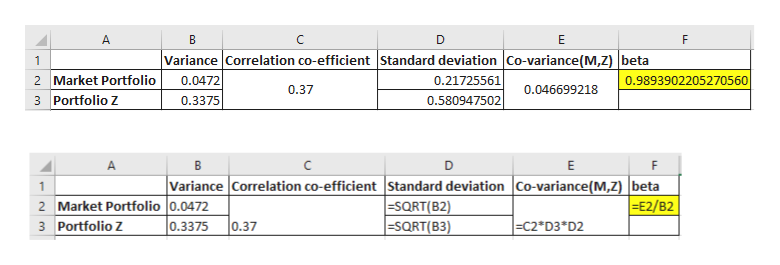# Suppose the risk-free rate is 5.1 percent and the market portfolio has an expected return of 11.8 percent. The market portfolio has a variance of .0472. Portfolio Z has a correlation coefficient with the market of .37 and a variance of .3375  According to the capital asset pricing model, what is the expected return on Portfolio Z?

Question
8 views
 Suppose the risk-free rate is 5.1 percent and the market portfolio has an expected return of 11.8 percent. The market portfolio has a variance of .0472. Portfolio Z has a correlation coefficient with the market of .37 and a variance of .3375

 According to the capital asset pricing model, what is the expected return on Portfolio Z?
check_circle

Step 1

Standard deviation of market portfolio and portfolio Z is calculated. Then covariance and beta of the portfolio Z is calculated as in the excel below:

Step 2help_outlineImage TranscriptioncloseA C Е F Variance Correlation co-efficient Standard deviation Co-variance(M,Z) beta 0.0472 0.21725561 2 Market Portfolio 0.9893902205270560 0.37 0.046699218 3 Portfolio Z 0.3375 0.580947502 в D E F Variance Correlation co-efficient Standard deviation Co-variance(M,Z) |beta SQRT(B2) =SQRT(B3) E2/B2 2 Market Portfolio 0.0472 0.3375 0.37 3 Portfolio Zz C2*D3 D2 co. fullscreen
Step 3

Using CAPM m...

### Want to see the full answer?

See Solution

#### Want to see this answer and more?

Solutions are written by subject experts who are available 24/7. Questions are typically answered within 1 hour.*

See Solution
*Response times may vary by subject and question.
Tagged in